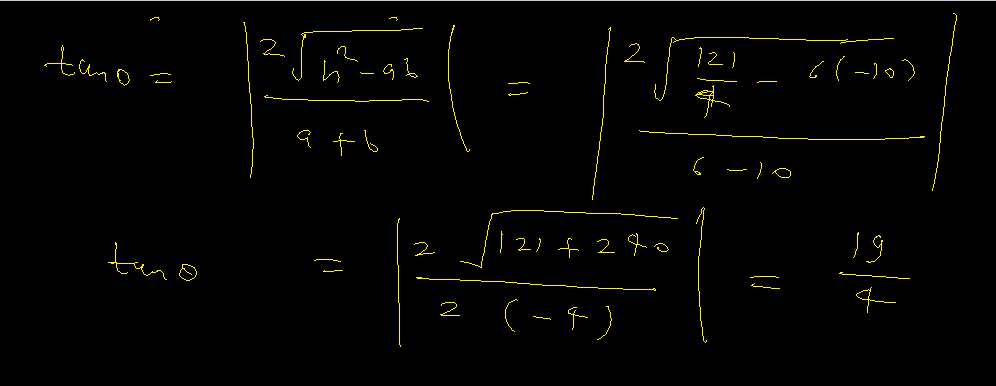×#### Thank you for registering.

One of our academic counsellors will contact you within 1 working day.

Click to Chat

1800-1023-196

+91-120-4616500

CART 0

• 0

MY CART (5)

Use Coupon: CART20 and get 20% off on all online Study Material

ITEM
DETAILS
MRP
DISCOUNT
FINAL PRICE
Total Price: Rs.

There are no items in this cart.
Continue Shopping
```
If the equation 6x^2-11xy-10y^2-19y+c=0 represents a pair of straight lines find their quarks. Also find the angle between the two lines.
If the equation 6x^2-11xy-10y^2-19y+c=0 represents a pair of straight lines find their quarks. Also find the angle between the two lines.

```
3 years agoDeepak Kumar Shringi
4404 Points
``````
2 years ago
```							we are given the equation of staright line 6x2-11xy-10y2-19y+c=0=> 6x2-11xy-10y2=19y-c=> 6x2-15xy+4xy-10y2=19y-c=> (3x+2y)(2x-5y)=19y-c      straight line will be of the form       3x+2y+c1=0 and 2x-5y+c2=0=>  2c1+3c2=0----------1=>  2c2-5c1= -19=>  c1c2=c      from equation 1 and 2 we get;      c1=3 and c2= -2      c1c2=c= -6      hence two lines will be 2x-5y-2=0 and 3x+2y+3=0
```
2 months ago
Think You Can Provide A Better Answer ?

## Other Related Questions on Analytical Geometry

View all Questions »### Course Features

• 731 Video Lectures
• Revision Notes
• Previous Year Papers
• Mind Map
• Study Planner
• NCERT Solutions
• Discussion Forum
• Test paper with Video Solution### Course Features

• 53 Video Lectures
• Revision Notes
• Test paper with Video Solution
• Mind Map
• Study Planner
• NCERT Solutions
• Discussion Forum
• Previous Year Exam Questions